Photo by Isaac Smith on Unsplash

# Identifying and Quantifying Trends in Time Series Data using the Mann-Kendall Test

In this article I discuss using Mann Kendall methods to automatically identify the trend in a time series.

This method will allow you to automatically 1) identify if a trend exists and 2) determine the strength of that trend using a statistical approach.

This will not decompose the time series into trend/seasonality/noise components, as there are methods to do that.

At first glance, determining the trend seems like a trivial problem. The problem becomes more complex when one has to determine the trend for hundreds of thousands of time series. This means visual inspection is not an option.

For my purpose, the method had to meet the following criteria:

1. Determine if a trend exists
2. Determine the strength/magnitude of the trend
3. Determine the direction of the trend (negative/positive)
4. Be distribution free: the metric should not rely on strong normality assumptions or the underlying values (e.g. the output for house prices should be equivalent for temperature if the strength and magnitudes are comparable)

# What is a trend?

A trend is defined as: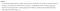Source: https://otexts.com/fpp2/tspatterns.html

Note that this doesn’t aim to quantify the stability or strength of the trend. It doesn’t answer whether a trend is positive or negative.

Trends are *kind of* easy to spot — visually.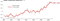An uptrend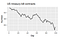A downtrend

And sometimes, not so much:?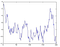Down-ish?

It wasn’t until I began peeling back the layers that I realized most of what is considered trend analysis is very arbitrary.

# Mann-Kendall Test

The Mann Kendall Test essentially covers all of the criteria I mentioned above. There are variations of this test that need to be applied when the data have a serial correlation, as is the case for many time series.

The original Mann Kendall test performs the following hypothesis test for a univariate time series:

The test statistic: `S` is defined as:

Where `sgn` is equal to

The variance is defined as:

With p being the number of “tied” groups, and t_j is the number of points in each group j.

Then, the S statistic is normalized with the following:

Given that I want to have a broadly applicable way of determining the trend in the time series, I created a “consensus” method that applies all relevant variations of the MK test to an input time series and then averages the trend (`-1` for negative, `0` for none, and `1` for positive.)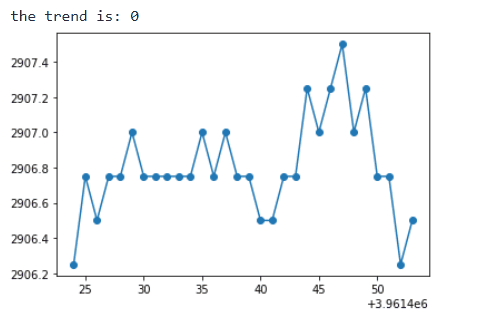Image by Author

It works!

The code used to generate this example is here:

Thanks for reading!

If you liked this article, you may also like:

Data Scientist